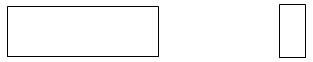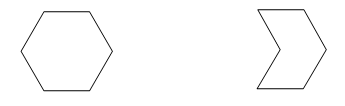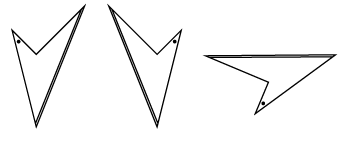# Congruence

Plane shapes which have the same shape and are the same size are called congruent.

For shapes to be congruent they must satisfy both the following conditions:

• matching (or corresponding) angles are equal
• matching (or corresponding) sides are equal in length.

These two shapes have matching angles equal but their matching sides are not equal and so they are not congruent. They are the same shape but not the same size.Non-congruent rectangles.

These two polygons have matching sides equal but their matching angles are not equal and so they are not congruent. They are different shapes even though the sides are the same size.Non-congruent hexagons.

Congruent shapes can be superimposed on each other by rotation, translation or reflection (or a combination of these transformations).All of these quadrilaterals are congruent.

Congruence can be a feature of any type of plane shape. Explore the link between congruent shapes and transformations further.

There are special conditions for congruent triangles.

## Congruent triangles

There are exactly four combinations of given angles and sides needed to uniquely describe a triangle.